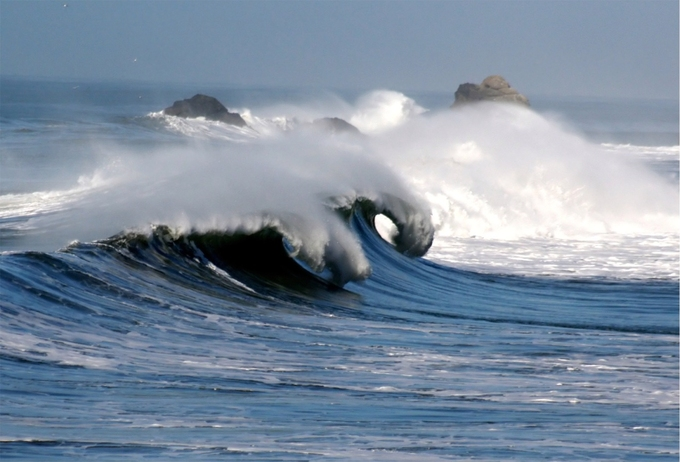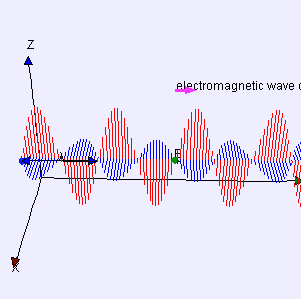Categories

# Energy Transportation

Waves transfer energy which can be used to do work.

Energy transportion is essential to waves. It is a common misconception that waves move mass. Waves carry energy along an axis defined to be the direction of propagation. One easy example is to imagine that you are standing in the surf and you are hit by a significantly large wave, and once you are hit you are displaced (unless you hold firmly to your ground!). In this sense the wave has done work (it applied a force over a distance). Since work is done over time, the energy carried by a wave can be used to generate power.Water Wave: Waves that are more massive or have a greater velocity transport more energy.

Similarly we find that electromagnetic waves carry energy. Electromagnetic radiation (EMR) carries energy—sometimes called radiant energy—through space continuously away from the source (this is not true of the near-field part of the EM field). Electromagnetic waves can be imagined as a self-propagating transverse oscillating wave of electric and magnetic fields.

EMR also carries both momentum and angular momentum. These properties may all be imparted to matter with which it interacts (through work). EMR is produced from other types of energy when created, and it is converted to other types of energy when it is destroyed. The photon is the quantum of the electromagnetic interaction, and is the basic “unit” or constituent of all forms of EMR. The quantum nature of light becomes more apparent at high frequencies (or high photon energy). Such photons behave more like particles than lower-frequency photons do.Electromagnetic Wave: Electromagnetic waves can be imagined as a self-propagating transverse oscillating wave of electric and magnetic fields. This 3D diagram shows a plane linearly polarized wave propagating from left to right.

In general, there is a relation of waves which states that the velocity (vv) of a wave is proportional to the frequency (ff) times the wavelength (λλ):

v=fλv=fλ

We also know that classical momentum pp is given by p=mvp=mv which relates to force via Newton’s second law: F=dpdtF=dpdt

EM waves with higher frequencies carry more energy. This is a direct result of the equations above. Since v∝fv∝f we find that higher frequencies imply greater velocity. If velocity is increased then we have greater momentum which implies a greater force (it gets a little bit tricky when we talk about particles moving close to the speed of light, but this observation holds in the classical sense).

Since energy is the ability of an object to do work, we find that for W=FdW=Fd a greater force correlates to more energy transfer. Again, this is an easy phenomenon to experience empirically; just stand in front of a faster wave and feel the difference!